Work Power Energy Exam1 and Problem Solutions

Work Power Energy Exams1 (Work) and  Problem Solutions

1.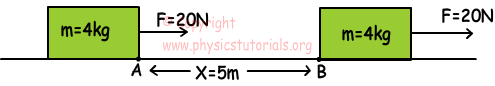In the picture given above F pulls a box having 4kg mass from point A to B. If the friction constant between surface and box is 0,3; find the work done by F, work done by friction force and work done by resultant force.

Work done by F;

WF=F.X=20.5=100 joule

Work done by friction force;

Wfriction=-Ff.X=-k.mg.X=-0,3.4.10.5=-60 joule

Work done by resultant force;

Wnet=Fnet.X=(F-Ff).X=(20-0,3.4.10)5

Wnet=40 joule

2. Applied force vs. position graph of an object is given below. Find the work done by the forces on the object.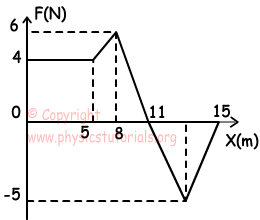Area under the graph gives us work done by the force.

Work done between 0-5m: W1=4.5=20 joule

Work done between 5-8m:

W2=(6+4)/2.3=15 joule

Work done between 8-11m:

W3= 6.3/2=9 joule

Work done between 11-15m:

W4=-5.4/2=-10 joule

Wnet=W1+W2+W3+W4=20+15+9+(-10) Wnet=34 joule

3.  Different forces are applied to three objects having equal masses. Forces pull objects to height h. Find the work done by the forces on objects and work done on gravity.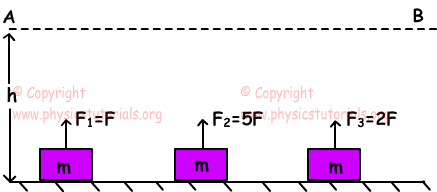W1=F.h, W2=5F.h,  W3=2F.h

Since masses of the objects are equal, and distance taken by the objects are equal, work done on gravity of three objects are equal.

4. In the picture given below, forces act on objects. Works done on objects during time t are W1, W2 and W3. Find the relation of the works.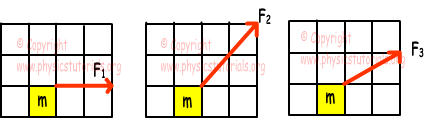Horizontal components of the applied forces are equal to each other. Masses of the objects are also equal. Thus, acceleration of the objects and distances taken are also equal.

Work done:

W=FX.X W1=W2=W3

5. A box having 2 kg mass, under the effect of forces F1, F2 and F3, takes distance 5m. Which ones of the forces do work.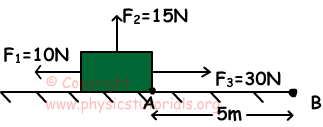Since box moves from point A to B, only F3 does work.

W3=F3.X

W3=30.5=150 joule

Author: